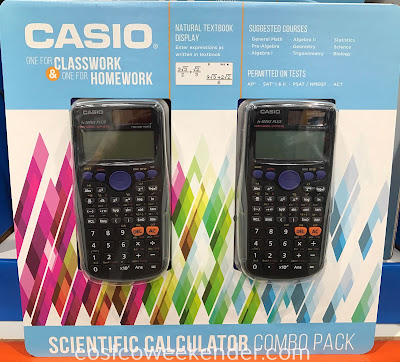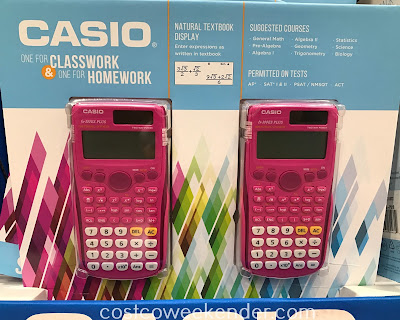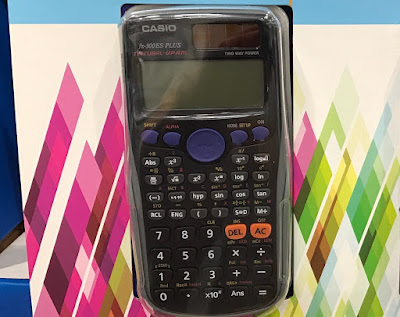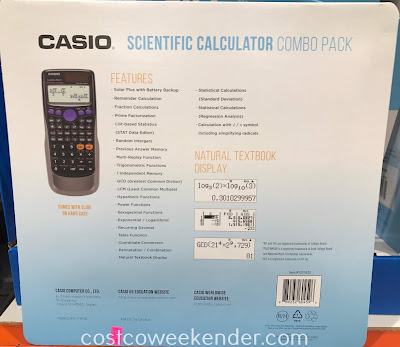# Casio Scientific Calculator fx-300ES PLUS

[Scroll down to view product info]

If your child needs a good calculator for school, then check out the Casio Scientific Calculator fx-300ES PLUS. A good calculator can make a huge difference on tests, homework, and other math problems. It's the perfect tool for high school algebra, geometry, trigonometry, etc. Don't be surprised if your teenager's grades go up with the Casio fx-300ES PLUS Scientific Calculator. The fx-300ES PLUS comes in a pack of two, so your child can use one for school and you can have one for the office or for home. This comes in handy when paying bills every month.Easily Get some homework done with the Casio Scientific Calculator fx-300ES PLUS

Here are the specs:

## Casio Scientific Calculator fx-300ES PLUS (2 pack) Product Info

Costco Item#: 1231822
Costco Price: \$14.99
Found at: Costco in Foster City, CA (1001 Metro Center Blvd.)
(price and availability may vary per Costco location)Combo pack includes 2 Casio fx-300ES PLUS calculators: for school and for homeTricky math problems just got easier with the Casio Scientific Calculator fx-300ES PLUSCasio fx-300ES PLUS Scientific Calculator: great for school, the office, or paying bills

Casio Scientific Calculator fx-300ES PLUS FX300ESPLUS (2 pack)

Key Features:
• Natural textbook display: enter expressions as written in textbook
• Suggested courses: general math, pre-algebra, algebra I, algebra II, geometry, trigonometry, statistics, science, biology
• Permitted on tests: AP, SAT I & II, PSAT/NMSQT, ACT
• 249 built-in functions
• Bonus 2nd calculator: one for classwork & one for homework
• Easy to use menu function
• Comes with slide on hard case
• Solar Plus with battery backup power
• Remainder calculation
• Fraction calculations
• Prime factorization
• List-based statistics (STAT Data Editor)
• Random integers
• Multi-replay function
• Trigonometric functions
• 1 independent memory
• GCD (Greatest Common Divisor)
• LCM (Least Common Multiple)
• Hyperbolic functions
• Power functions
• Sexagesimal functions
• Exponential/logarithmic
• Recurring decimal
• Table function
• Coordinate conversion
• Permutation/combination
• Statistical calculations: standard deviation, regression analysis
• Calculation with ÷/n symbol including simplifying radicals